StatLect

# Bayes' rule

Bayes' rule, named after the English mathematician Thomas Bayes, is a rule for computing conditional probabilities.## The rule

A formal statement of Bayes' rule follows.

Proposition Letandbe two events. Denote their probabilities byandand suppose that bothand. Denote bythe conditional probability ofgivenand bythe conditional probability ofgiven. Bayes' rule states thatProof

By the conditional probability formula, we have thatandThe second formula can be re-arranged as follows:which is then plugged into the first formula, so as to obtainThe following example shows how Bayes' rule can be applied in a practical situation.

Example We built a robot that can detect defective items produced in our factory. If an item is defective, it is spotted with 98% probability by the robot. When an item is not defective, the robot will not signal any defect with 99% probability. We draw an item at random from a production lot in which 0,1% of items are defective. If the robot tells us that the drawn item is defective, what is the probability that the robot is right? In probabilistic terms, what we know about this problem can be formalized as follows: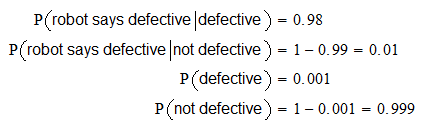Furthermore, the unconditional probability that the robot signals a defective item can be derived using the law of total probability: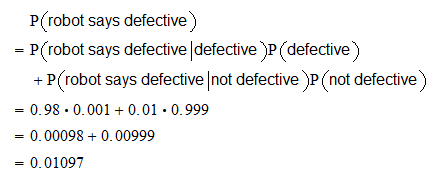Therefore, Bayes' rule gives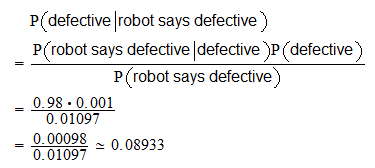Therefore, even if the robot is conditionally very accurate, the unconditional probability that the robot is right when he says that an item is defective is less than 10 per cent!

## Terminology

The quantities involved in Bayes' ruleoften take the following names:

1.is called prior probability or, simply, prior;

2.is called conditional probability or likelihood;

3.is called marginal probability;

4.is called posterior probability or, simply, posterior.

## Solved exercises

Below you can find some exercises with explained solutions.

### Exercise 1

There are two urns containing colored balls. The first urn contains 50 red balls and 50 blue balls. The second urn contains 30 red balls and 70 blue balls. One of the two urns is randomly chosen (both urns have probabilityof being chosen) and then a ball is drawn at random from one of the two urns. If a red ball is drawn, what is the probability that it comes from the first urn?

Solution

In probabilistic terms, what we know about this problem can be formalized as follows:The unconditional probability of drawing a red ball can be derived using the law of total probability:By using Bayes' rule, we obtain### Exercise 2

An economics consulting firm has created a model to predict recessions. The model predicts a recession with probability 80% when a recession is indeed coming and with probability 10% when no recession is coming. The unconditional probability of falling into a recession is 20%. If the model predicts a recession, what is the probability that a recession will indeed come?

Solution

What we know about this problem can be formalized as follows: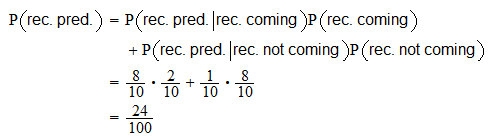The unconditional probability of predicting a recession can be derived by using the law of total probability:Bayes' rule implies### Exercise 3

Alice has two coins in her pocket, a fair coin (head on one side and tail on the other side) and a two-headed coin. She picks one at random from her pocket, tosses it and obtains head. What is the probability that she flipped the fair coin?

Solution

What we know about this problem can be formalized as follows:The unconditional probability of obtaining head can be derived by using the law of total probability: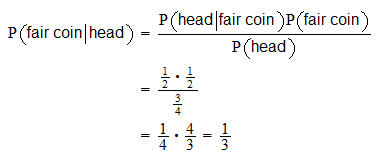With Bayes' rule, we obtainThe book

Most of the learning materials found on this website are now available in a traditional textbook format.

Glossary entries
Share